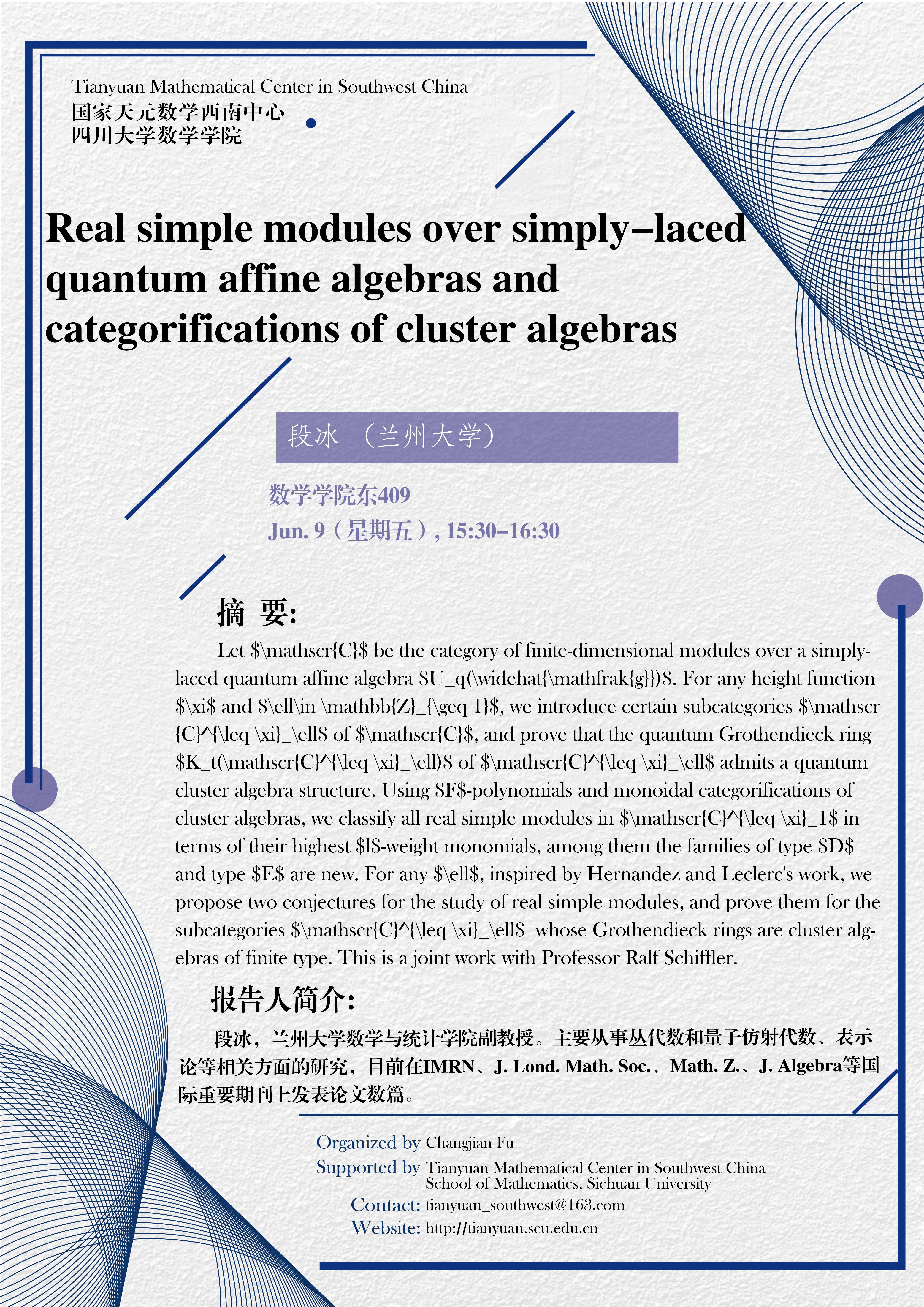Real simple modules over simply-laced quantum affine algebras and categorifications of cluster algebras

Let $\mathscr{C}$ be the category of finite-dimensional modules over a simply-laced quantum affine algebra $U_q(\widehat{\mathfrak{g}})$. For any height function $\xi$ and $\ell\in \mathbb{Z}_{\geq 1}$, we introduce certain subcategories $\mathscr{C}^{\leq \xi}_\ell$ of $\mathscr{C}$, and prove that the quantum Grothendieck ring $K_t(\mathscr{C}^{\leq \xi}_\ell)$ of $\mathscr{C}^{\leq \xi}_\ell$ admits a quantum cluster algebra structure. Using $F$-polynomials and monoidal categorifications of cluster algebras, we classify all real simple modules in $\mathscr{C}^{\leq \xi}_1$ in terms of their highest $l$-weight monomials, among them the families of type $D$ and type $E$ are new. For any $\ell$, inspired by Hernandez and Leclerc's work, we propose two conjectures for the study of real simple modules, and prove them for the subcategories $\mathscr{C}^{\leq \xi}_\ell$  whose Grothendieck rings are cluster algebras of finite type. This is a joint work with Professor Ralf Schiffler.